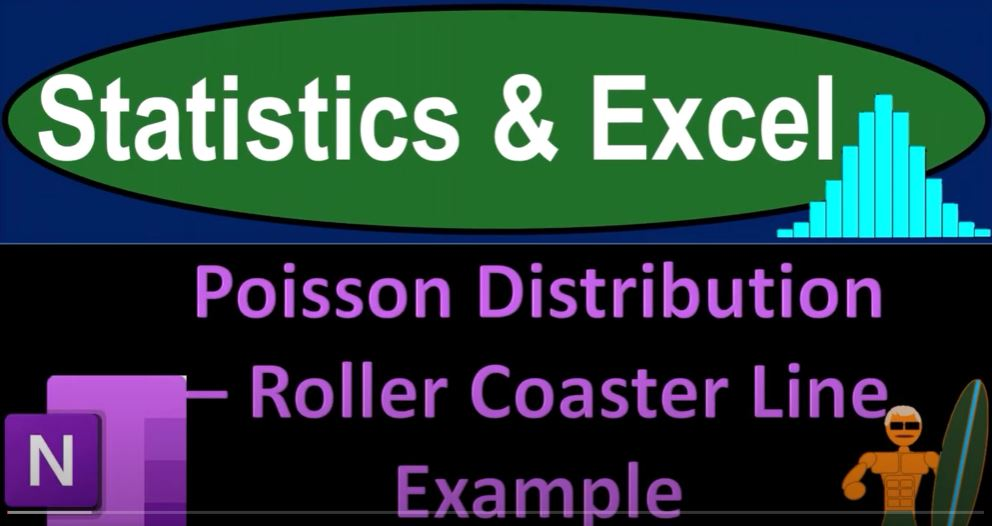## Poisson Distribution – Roller Coaster Line Example 1537 Statistics & Excel

In the world of statistics and data analysis, understanding the distribution of data is a fundamental concept. We often use mathematical formulas and visual representations to make sense of datasets. In this blog post, we will delve into the world of distribution using a roller coaster line example. We’ll explore how statistics and Excel can help us gain insights into data and make predictions.

Why Distribution Matters: Before we jump into the roller coaster example, let’s quickly review why understanding distribution is crucial. When working with data, we want to know how it’s spread out. Are values clustered around a central point, or do they vary widely? Distribution helps us answer these questions.

Distribution Types: In previous presentations, we discussed various distribution types. You might recall terms like “skewed to the right” or “skewed to the left.” These descriptions help us understand how data is distributed. Now, we’re focusing on a particular distribution – the Poisson distribution.

The Roller Coaster Line Example: Imagine waiting in line for a roller coaster ride. We want to analyze how many people arrive within a one-minute timeframe. “x” represents the number of arrivals during one minute. The “mean” (average) is a crucial statistic when working with Excel functions, which we’ll explore shortly.

Data Generation in Excel: To analyze this example, we can use Excel. We generate random numbers that simulate arrivals within a one-minute interval. It’s important to note that we provide the mean to Excel to create these random numbers following a Poisson distribution. These generated numbers represent how many people arrive in each one-minute time interval.

Data Binning: Once we have our data, we group it into bins or buckets. Each bin represents the number of arrivals (e.g., 0, 1, 2, etc.). We then count how many times each number of arrivals occurred. In Excel, you can use the “FREQUENCY” function to do this. It’s a more reliable choice than “COUNTIF” because it handles fractional results better.

Calculating Mean and Variance: We calculate the mean (average) of the data using Excel’s “AVERAGE” function. The variance is an essential statistic too. We compute both the population and sample variances. If the mean and variance are similar, it’s an indicator that the data might follow a Poisson distribution.

Visualizing the Data: Visual representation is vital. We create a frequency distribution plot to observe the data’s shape. The Poisson distribution is typically skewed to the right, so our plot should reflect that shape.

Comparing Data and Poisson Distribution: Now, we compare our actual data with the Poisson distribution. We use the Poisson distribution formula, plugging in the mean, to estimate the probabilities of different arrival counts. If our data closely matches the Poisson distribution, we can use it to make predictions about future line arrivals.

Calculating Probabilities: We calculate probabilities of specific scenarios, like the likelihood of having fewer than three arrivals within a minute. Excel’s “POISSON.DIST” function comes in handy for these calculations.

Graphical Representation: Finally, we visualize the Poisson distribution we calculated alongside our data. While they won’t match exactly, a close alignment suggests that the Poisson distribution can be a valuable tool for predicting future arrivals in our roller coaster line.

Conclusion: Understanding distribution is essential for data analysis. In this roller coaster line example, we’ve seen how statistics and Excel can help us explore and leverage the Poisson distribution to make predictions. Whether you’re managing roller coaster lines or working with other datasets, these techniques can provide valuable insights and help you plan for the future.